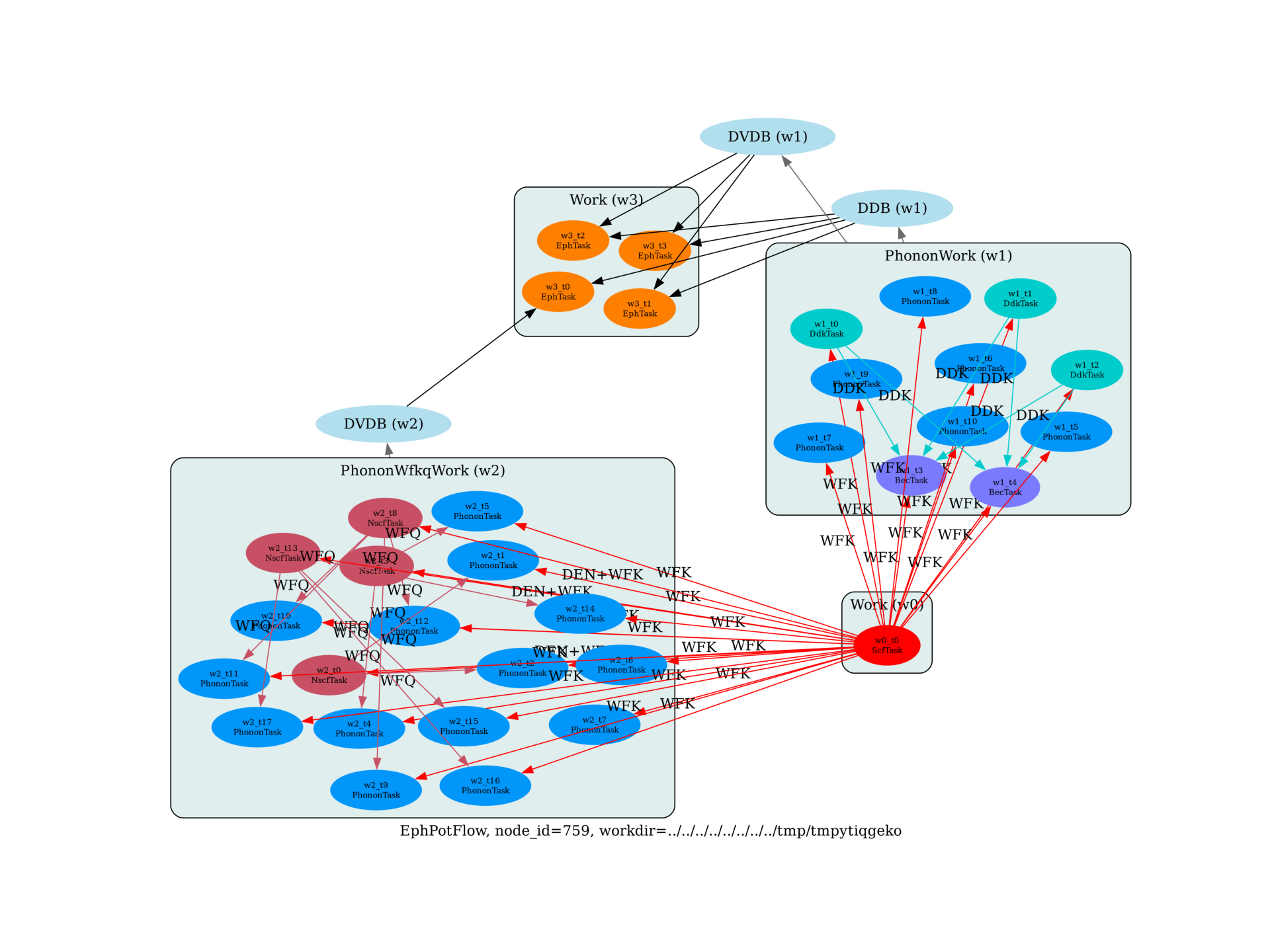# Flow to compute e-ph scattering potentials¶

This example shows how to compute e-ph scattering potentials along a q-path, merge the POT files in the DVDB file and use the DVDB and the DDB file to analyze the average over the unit cell of the periodic part as a function of qOut:

/Users/gmatteo/git_repos/pymatgen/pymatgen/util/plotting.py:550: UserWarning: Matplotlib is currently using agg, which is a non-GUI backend, so cannot show the figure.
plt.show()


import sys
import os
import abipy.abilab as abilab
import abipy.data as abidata

from abipy import flowtk

def make_scf_input(ngkpt):
"""
This function constructs the input file for the GS calculation:
"""
structure = dict(
angdeg=3*[60.0],
acell=3*[7.1992351952],
natom=2,
ntypat=2,
typat=[1, 2],
znucl=[31, 15],
xred=[
0.0000000000, 0.0000000000, 0.0000000000,
0.2500000000, 0.2500000000, 0.2500000000,
])

pseudos = abidata.pseudos("Ga.oncvpsp", "P.psp8")
gs_inp = abilab.AbinitInput(structure, pseudos=pseudos)

gs_inp.set_vars(
nband=8,
ecut=20.0,   # Too low
ngkpt=ngkpt,
nshiftk=1,
shiftk=[0, 0, 0],
tolvrs=1.0e-8,
nstep=150,
)

return gs_inp

def build_flow(options):
"""
Create a Flow for phonon calculations. The flow has two works.

The first work contains a single GS task that produces the WFK file used in DFPT
Then we have multiple Works that are generated automatically
in order to compute the dynamical matrix on a [2, 2, 2] mesh.
Symmetries are taken into account: only q-points in the IBZ are generated and
for each q-point only the independent atomic perturbations are computed.
"""
# Working directory (default is the name of the script with '.py' removed and "run_" replaced by "flow_")
if not options.workdir:
options.workdir = os.path.basename(sys.argv).replace(".py", "").replace("run_", "flow_")

# Use 2x2x2 both for k-mesh and q-mesh
# Build input for GS calculation
scf_input = make_scf_input(ngkpt=(2, 2, 2))

# Create flow to compute all the independent atomic perturbations
# corresponding to a [4, 4, 4] q-mesh.
# Electric field and Born effective charges are also computed.
from abipy.flowtk.eph_flows import EphPotFlow
ngqpt = (2, 2, 2)

qpath_list = [
+0.10000,  +0.10000,  +0.10000,  # L -> G
+0.00000,  +0.00000,  +0.00000,  # $\Gamma$
+0.10000,  +0.00000,  +0.10000,  # G -> X

#+0.50000,  +0.50000,  +0.50000,  # L
#+0.00000,  +0.00000,  +0.00000,  # $\Gamma$
#+0.50000,  +0.00000,  +0.50000,  # X
#+0.50000,  +0.25000,  +0.75000,  # W
#+0.37500,  +0.37500,  +0.75000,  # K
#+0.00000,  +0.00000,  +0.00000,  # $\Gamma$
#+0.50000,  +0.25000,  +0.75000,  # W
#+0.62500  +0.25000  +0.62500  # U
#+0.50000  +0.25000  +0.75000  # W
#+0.50000  +0.50000  +0.50000  # L
#+0.37500  +0.37500  +0.75000  # K
#+0.62500  +0.25000  +0.62500  # U
#+0.50000  +0.00000  +0.50000  # X
]

# Use small ndivsm to reduce computing time.
flow = EphPotFlow.from_scf_input(options.workdir, scf_input,
ngqpt, qpath_list, ndivsm=2, ddk_tolerance={"tolwfr": 1e-12},
with_becs=True)

return flow

# This block generates the thumbnails in the Abipy gallery.
# You can safely REMOVE this part if you are using this script for production runs.
__name__ = None
import tempfile
options = flowtk.build_flow_main_parser().parse_args(["-w", tempfile.mkdtemp()])
build_flow(options).graphviz_imshow()

@flowtk.flow_main
def main(options):
"""
This is our main function that will be invoked by the script.
flow_main is a decorator implementing the command line interface.
Command line args are stored in options.
"""
return build_flow(options)

if __name__ == "__main__":
sys.exit(main())


Total running time of the script: ( 0 minutes 5.054 seconds)

Gallery generated by Sphinx-Gallery